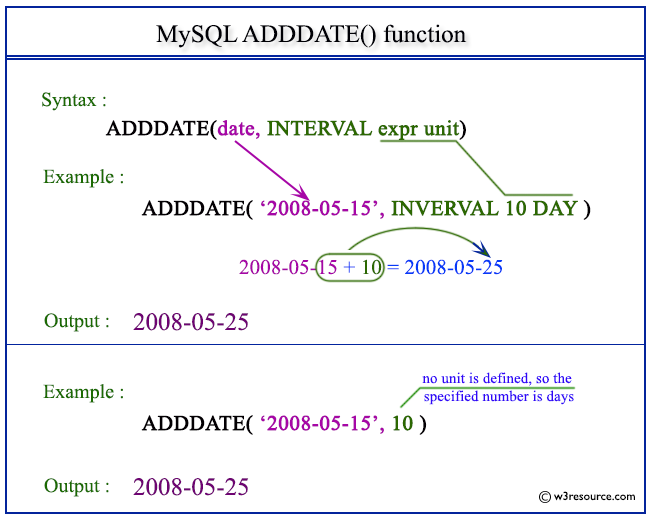The MySQL ADDDATE() function is used to add a specified time interval to a given date and return the resulting date value.

The common uses of MySQL ADDDATE() function -

• Date Arithmetic: The ADDDATE() function can be used to perform arithmetic operations on dates, such as adding or subtracting days, weeks, months, or years. This can be useful in a variety of applications, such as calculating due dates, scheduling tasks, or tracking time-based events.

• Date Calculations: The ADDDATE() function can be used to perform complex date calculations, such as finding the difference between two dates or calculating the age of a person or object based on a given date.

Syntax:

```ADDDATE(date, INTERVAL expr unit), ADDDATE(expr,days)
```

Arguments:

Name Description
date A date value.
INTERVAL Keyword.
expr A date or datetime expression or a number.
unit An unit, described in the following table.

Syntax Diagram:MySQL Version: 8.0

The following table shows the expected form of the "expr" argument for each unit value.

Unit Value Expected expr Format
MICROSECOND MICROSECONDS
SECOND SECONDS
MINUTE MINUTES
HOUR HOURS
DAY DAYS
WEEK WEEKS
MONTH MONTHS
QUARTER QUARTERS
YEAR YEARS
SECOND_MICROSECOND 'SECONDS.MICROSECONDS'
MINUTE_MICROSECOND 'MINUTES:SECONDS.MICROSECONDS'
MINUTE_SECOND 'MINUTES:SECONDS'
HOUR_MICROSECOND 'HOURS:MINUTES:SECONDS.MICROSECONDS'
HOUR_SECOND 'HOURS:MINUTES:SECONDS'
HOUR_MINUTE 'HOURS:MINUTES'
DAY_MICROSECOND 'DAYS HOURS:MINUTES:SECONDS.MICROSECONDS'
DAY_SECOND 'DAYS HOURS:MINUTES:SECONDS'
DAY_MINUTE 'DAYS HOURS:MINUTES'
DAY_HOUR 'DAYS HOURS'
YEAR_MONTH 'YEARS-MONTHS'Following code shows how to use the ADDDATE() function in MySQL to add days, months, or years to a date:

``````-- Add 7 days to the date '2019-04-28'

-- Add 3 months to the date '2019-04-28'

-- Add 2 years to the date '2019-04-28'
``````

Output:

```ADDDATE('2019-04-28', INTERVAL 7 DAY)
2019-05-05
2019-07-28
2021-04-28
```

In each of the above examples, the ADDDATE() function adds a specified number of days, months, or years to the original date value. It returns the resulting date value.

Use negative values to subtract days, months, or years from a date using ADDDATE() function

``````-- Subtract 7 days from the date '2019-04-28'

-- Subtract 3 months from the date '2019-04-28'

-- Subtract 2 years from the date '2019-04-28'
```
```

Output:

```ADDDATE('2019-04-19', INTERVAL -7 DAY)
2019-04-12
2019-01-28
2017-04-28
```

In each of the above examples, the INTERVAL value is negative, indicating that the specified time interval should be subtracted from the original date value.

How to use variables or parameters in MySQL's ADDDATE() function?

We can use variables or parameters in MySQL's ADDDATE() function. This is useful if we need to add a dynamic number of days, months, or years to a date. In addition, you can use the result of a subquery or a user-defined variable as input. Here's an example of how to use a variable in the ADDDATE() function:

Using a variable in the ADDDATE() function:

``````SET @num_days = 17;
```
```

Output:

```ADDDATE('2016-05-01', INTERVAL @num_days DAY)
2016-05-18
```

In the above example, we are setting the variable @num_days to 17, and then using it in the ADDDATE() function to add 17 days to the date '2016-05-01'.

Using a parameter in a stored procedure:

``````DELIMITER //
CREATE PROCEDURE add_days(IN date_val DATE, IN num_days INT)
BEGIN
END//

```
```

Output:

```ADDDATE(date_val, INTERVAL num_days DAY)
2016-05-18```

In the above code, we create a stored procedure called "add_days" that takes two input parameters: date_val and num_days. The ADDDATE() function is used in the stored procedure to add num_days to date_val, and the resulting date is returned. When the procedure is called with the values '2016-05-01' and 17, it will return the date '2016-05-18'.

How to handle date and time zones when using MySQL's ADDDATE() function?

To convert a date to a specific time zone in MySQL, we can use the CONVERT_TZ() function. Here's an example::

``````SELECT ADDDATE(CONVERT_TZ('2016-05-01 12:00:00', '+00:00', '+06:00'), INTERVAL 15 DAY);
```
```

Output:

```ADDDATE(CONVERT_TZ('2016-05-01 12:00:00', '+00:00', '+06:00'), INTERVAL 15 DAY)
2016-05-16 18:00:00
```

In the said example, we convert the date '2016-05-01 12:00:00' from UTC (+00:00) to United States Central Standard Time (+06:00) using the CONVERT_TZ() function. We add 15 days to this date using the ADDDATE() function.

Note: The CONVERT_TZ() function takes three arguments: the original date, the time zone to convert from, and the time zone to convert to. You should substitute the appropriate time zones for your scenario.

How to format MySQL's ADDDATE() function output?

When using the ADDDATE() function in MySQL, the resulting date is returned in the default YYYY-MM-DD format. However, we can format the function output using the DATE_FORMAT() function. Here are some examples of how to format the ADDDATE() output in MySQL:

``````SELECT DATE_FORMAT(ADDDATE('2016-05-01', INTERVAL 14 DAY), '%m/%d/%Y');
SELECT DATE_FORMAT(ADDDATE('2016-05-01', INTERVAL 14 DAY), '%m-%d-%Y');
SELECT DATE_FORMAT(ADDDATE('2016-05-01', INTERVAL 14 DAY), '%d/%m/%Y');
SELECT DATE_FORMAT(ADDDATE('2016-05-01', INTERVAL 14 DAY), '%Y-%m-%d');
SELECT DATE_FORMAT(ADDDATE('2016-05-01', INTERVAL 14 DAY), '%d-%b-%Y');
SELECT DATE_FORMAT(ADDDATE('2016-05-01', INTERVAL 14 DAY), '%W, %M %e, %Y');
```
```

Output:

```DATE_FORMAT(ADDDATE('2016-05-01', INTERVAL 14 DAY), '%m/%d/%Y')
05/15/2016
05-15-2016
15/05/2016
2016-05-15
15-May-2016
DATE_FORMAT(ADDDATE('2016-05-01', INTERVAL 14 DAY), '%W, %M %e, %Y')
Sunday, May 15, 2016
```

All Date and Time Functions :